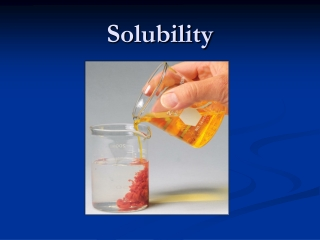DownloadDownload PresentationSolubility

# Solubility

Download Presentation## Solubility

- - - - - - - - - - - - - - - - - - - - - - - - - - - E N D - - - - - - - - - - - - - - - - - - - - - - - - - - -
##### Presentation Transcript

1. Solubility

2. Solubility “Insoluble” salts are governed by equilibrium reactions, and are really sparingly soluble. There is a dynamic equilibrium between the solvated ions and the crystalline solid.

3. Solubility

4. Solubility The extent to which a substance dissolves is its solubility. Solubility, as with all equilibria, is highly temperature dependent. Solubility may be expressed as grams of compound/100 mL of H2O, or as molarity, M.

5. Solubility Conventions The equilibrium constants for solubility are based on the insoluble solid appearing on the left side of the reaction, with the solvated ions on the right side. PbI2(s) ↔ Pb2+(aq) + 2I-(aq) Ksp = [Pb2+][ I- ]2 = 1.4 x 10-8 @25oC sp stands for solubility product.

6. Solubility The molar solubility can be calculated from the value of Ksp (and vice versa). Since salts have differing numbers of cations and anions, you cannot look at a table of Ksp values and determine which compounds have the greater solubility.

7. Ksp Values

8. Problem • Calcium phosphate has a Ksp of 1.3 x 10-32. Calculate the molar solubility of calcium phosphate.

9. The Common- Ion Effect The solubility of a sparingly soluble salt will be lower if one of the ions of the salt is provided by an external source. Silver iodide is sparingly soluble. Its solubility in water will be greater than in a solution of aqueous NaI. The additional iodide ion will cause the AgI to precipitate out of solution.

10. The Common- Ion Effect

11. pH and Solubility Since most anions are weak bases, solubility is highly pH dependent. The basic anion may become protonated in acidic solutions, and thus the “insoluble” salt will dissolve.

12. pH and Solubility For example, consider the solubility of CuS. Ksp=8.5 x 10-45. Sufide ion is the conjugate base of HS-, a weak acid with a Ka of 1.3 x 10-13. This means that sulfide ion is a relatively strong weak base, and will accept protons.

13. pH and Solubility Solubility Reaction: CuS(s) ↔ Cu2+(aq) + S2-(aq) Ksp= 8.5 x 10-45 Reaction of Sulfide with Acid: S2-(aq) + H3O+(aq) ↔ HS-(aq) + H2O(l) Kb = Kw/Ka = (1.0 x 10-14)/1.3 x 10-13 = 7.7 x 10-2 Since sulfide is basic, CuS will dissolve in acidic solution.

14. pH and Solubility Since sulfide is basic, CuS will dissolve in acidic solution. As acid is added, this reaction is shifted towards the right. S2-(aq) + H3O+(aq) ↔ HS-(aq) + H2O(l)

15. pH and Solubility Since sulfide is basic, CuS will dissolve in acidic solution. As acid is added, this reaction is shifted towards the right. S2-(aq) + H3O+(aq) ↔ HS-(aq) + H2O(l)

16. pH and Solubility Since sulfide is basic, CuS will dissolve in acidic solution. As acid is added, this reaction is shifted towards the right. S2-(aq) + H3O+(aq) ↔ HS-(aq) + H2O(l) The decrease in [S2-] shifts this reaction toward the right so as to make more sulfide. CuS(s) ↔ Cu2+(aq) + S2-(aq)

17. pH and Solubility CuS(s) ↔ Cu2+(aq) + S2-(aq) The result is a large increase in solubility of CuS in acidic solutions.

18. Problem: pH and Solubility • Calculate the solubility of Fe(OH)2 in buffers of pH=3.00 and pH= 11.00. Ksp for Fe(OH)2 =1.8 x 10-15.

19. Predicting Precipitation Reactions If the ions which may form a precipitate come from two different sources (the cation comes from one soluble salt, the anion from another), calculate the value of Q.

20. Predicting Precipitation Reactions • Will a precipitate form if 10.0 mL of 0.010M AgNO3 is added to 20.0 mL of 0.10M Na2SO4? • Determine the “insoluble” product. • Calculate the concentration of each ion of the “insoluble” product. • Calculate Q and compare the value to Ksp.

21. Ksp Values

22. Selective Precipitation Mixtures of metal ions in aqueous solution are often separated by selective precipitation. A solution containing an anion that will only precipitate one of the metals while leaving the others in solution is used.

23. Selective Precipitation • A solution contains 0.25M Ni(NO3)2 and 0.25M Cu(NO3)2. Can the metal ions be separated by slowly adding Na2CO3? (Assume that for successful precipitation 99% of the metal ion must be precipitated before the other metal ion begins to precipitate, and assume no volume change on addition of sodium carbonate.) Ksp for NiCO3 =1.4 x 10-7, Ksp for CuCO3 =2.5 x 10-10.

24. Complex Ion Equilibria A complex ion contains a central metal ion bound to one or more ligands. A ligand is a molecule or ion that acts as a Lewis base in bonding to the metal ion. Ag+(aq) + 2NH3(aq) Ag(NH3)2+(aq)

25. Complex Ion Equilibria The equilibrium reactions are associated with an equilibrium constant called a formation constant (Kf). Ag+(aq) + 2NH3(aq) ↔Ag(NH3)2+(aq) Kf =[Ag(NH3)2+]/[Ag+][NH3]2

26. Formation Constants Most formation constants are quite large, indicating that the reactions go very nearly to completion. As a result, metal metal ion salts with low solubility can be easily dissolved if the proper ligand is introduced into solution.

27. Complex Formation Silver chloride is quite insoluble, but the addition of ammonia will form a stable complex ion, and cause the silver chloride to dissolve.

28. Ksp for AgCl = 1.77 x 10-10 Kf for Ag(NH3)2+ = 1.7 x 107 As a result, silver chloride dissolves when aqueous ammonia is added.